# Transformations. In mathematics or computer graphics, picking a transformation at random is almost certain to distort objects as they move

 Page 1/61 Date 26.04.2018 Size 5.82 Mb.
Transformations of the plane
In order to automate the process of animation a computer needs a formula for computing the new location of a point. In other words, the computer requires a special kind of function which takes in the coordinates of a point, and spits out the coordinates of a new, transformed point. In math, such functions are called transformations.
In mathematics or computer graphics, picking a transformation at random is almost certain to distort objects as they move. Consequently, for computer animation, the transformations we are most interested in are the distortion-free ones, which correspond to motion in the physical world. Such transformations are called isometries, coming from the Greek, meaning roughly equal measures.
Isometries of the plane
Isometries create patterns that help us organize our world conceptually. These patterns occur in nature, and are invented by artists, craftspeople, musicians, choreographers, and mathematicians.

Plane isometry involves moving all points around the plane so that their positions relative to each other remain the same, although their absolute positions may change. Isometries preserve distances, angles, sizes, and shapes. They are “rigid motions”.

In mathematics, the idea of isometry gives us a precise way to think about this subject. We will talk about plane isometries, those that take place on a flat plane, but the ideas generalize to spatial isometries too.

Rotation:To rotate an object means to turn it around. Every rotation has a center and an angle.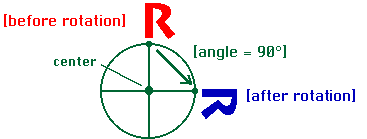Translation:To translate an object means to move it without rotating or reflecting it. Every translation has a direction and a distance.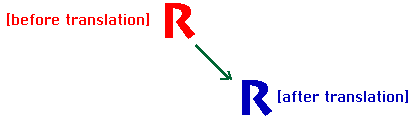Reflection:To reflect an object means to produce its mirror image. Every reflection has a mirror line. A reflection of an "R" is a backwards "R". (But we can consider every reflection to be a special case of a glide reflection).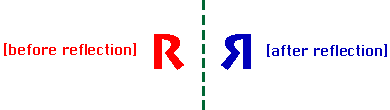Glide Reflection:A glide reflection combines a reflection with a translation along the direction of the mirror line. Glide reflections are the only type of symmetry that involve more than one step.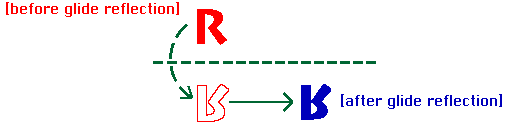The identity isometry: It can be considered a fifth kind. Alternatively, we can consider the identity a special case of a translation, and also a special case of a rotation.

We really have only three categories: rotations, translations, and glide reflections, which are mutually exclusive except for the identity.

Recognition of isometries
We can recognize which of these isometries we have according to whether it preserves orientation or not (direct and opposite isometries), and whether it has at least one fixed point or not, as shown in the following table (omitting the identity).
 Preserves orientation? Yes No Fixed point? Yes Rotation Reflection No Translation Glide reflection

Compositions of isometries
What happens when you do one isometry followed by another? Obviously the result is another isometry, since neither transformation introduces any distortion.
Two rotations of the same centre produce a new rotation with the same centre and with angle equal to the adition of the angles.

Two translations produce a new translation in which the vector is equal to the adition of the vectors.
If we do one reflection followed by another, notice the result will have to be orientation-preserving; that suggests a product of reflections should be either a translation or a rotation.

(depending on whether or not the mirror lines of the two relfections cross; if the mirror lines cross the intersection point will be a fixed point of the product).

We also can emulate the effect of translation by doing two reflections.

We can emulate the effect of a glide reflection by doing three reflections.

As a result, we can really just focus on the behavior of reflections, since the other three types of isometry can be built up from reflections.
Any isometry of the plane is a translation, rotation, reflection or glide reflection; no matter how you compose them, you will always end up with another isometry on this list.

N-fold rotational symmetry
Rotational symmetry of order n, also called n-fold rotational symmetry, or discrete rotational symmetry of nth order, with respect to a particular point (in 2D) means that rotation by an angle of 360°/n does not change the object.

The notation for n-fold symmetry is Cn or simply "n".

Examples: n = 2, 180°: rectangle, rhombus, letters Z, N, S, yin and yang symbol

n = 3, 120°: triskelion

n = 4, 90°: swastika

n = 6, 60°: raelian symbol, new version

More examples:

Nederlandse Spoorwegen (Dutch Railways)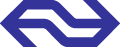N=2

Traffic sign roundabout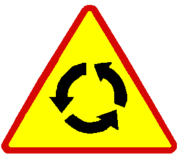N=3

The flag of Nazi Germany and the NSDAP, with swastika.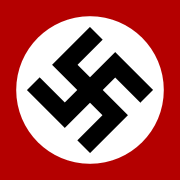N=4

White circle in Syrian Social Nationalist Party flagN=4

The swastika in decorative Hindu form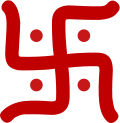N=4

The Raëlian symbol, before and after 1991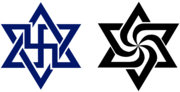N=2, N=6
Exercises:
Classify all the capital letters in English (in their simplest forms) according to their symmetries. For example, "A" has a reflection in a vertical line, and "R" has no symmetry.
Make a pattern by starting with an asymmetric shape and repeating a single translation over and over. That is, decide on a direction and distance for your translation (for example, 5 cm to the right). Translate your shape 5 cm to the right, then translate the new one 5 cm to the right, etc. Also translate the original letter 5 cm. to the left, etc. Did you get any other types of isometries (reflections, glide reflections, or rotations) in the process?
Make a symmetric pattern by starting with an asymmetric shape and repeating a single glide reflection over and over (also glide it backwards). That is, pick a reflection line and a translation in a direction parallel to the reflection line. Keep applying the same glide reflection to the new shapes that you generate until you run out of paper. Did you get any other types of isometries (reflections, translations, or rotations) in the process?
On a piece of paper, draw a letter R (call it R1) and two parallel lines (L1 and L2) about an inch apart. Reflect the R across L1 and call it R2. Reflect R2 across L2 and call it R3.

a) How is R3 related to R1? (by which type of isometry?)

b) Continue your pattern by reflecting the new Rs across L1 and L2. Would this be an infinite pattern if you had an infinitely large piece of paper?

c) What isometries does your pattern have besides reflections across L1 and L2?

Repeat the previous activity using lines that are not parallel but intersect at a 45-degree angle.

a) Now how is R3 related to R1?

b) Continue your pattern by reflecting the new Rs across L1 and L2. Would this be an infinite pattern if you had an infinitely large piece of paper?

c) What isometries does your pattern have besides reflections across L1 and L2?

Do you remember making strings of paper dolls or snowflakes by cutting a strip of folded paper? Adapt one of these activities so that it explicitly talks about isometries.
Consider patterns generated by reflections across two intersecting lines, but use other angles. For example, what if the lines formed an angle of 111 degrees, or 60 degrees? If you can, find a general pattern that will predict results for any angle.

Symmetry in the arts and crafts
A